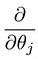# Machine Learning week 1: Cost Function, Gradient Descent and Univariate Linear Regression

I have started doing Andrew Ng’s popular machine learning course on Coursera. The first week covers a lot, at least for someone who hasn’t touched much calculus for a few years

• Cost Functions (mean difference squared)
• Linear Regression

These three topics were a lot to take in. I’ll talk about each in detail, and how they all fit together, with some python code to demonstrate.

Edit May 4th: I published a follow up focusing on how the Cost Function works here, including an intuition, how to calculate it by hand and two different Python implementations. I can do gradient descent and then bring them together for linear regression soon.

## Model Representation

First, the goal of most machine learning algorithms is to construct a model: a hypothesis that can be used to estimate Y based on X. The hypothesis, or model, maps inputs to outputs. So, for example, say I train a model based on a bunch of housing data that includes the size of the house and the sale price. By training a model, I can give you an estimate on how much you can sell your house for based on it’s size. This is an example of a regression problem — given some input, we want to predict a continuous output.

The hypothesis is usually presented as

The theta values are the parameters.

Some quick examples of how we visualize the hypothesis:

This yields h(x) = 1.5 + 0x. 0x means no slope, and y will always be the constant 1.5. This looks like:

The goal of creating a model is to choose parameters, or theta values, so that h(x) is close to y for the training data, x and y. So for this data

`x = [1, 1, 2, 3, 4, 3, 4, 6, 4]y = [2, 1, 0.5, 1, 3, 3, 2, 5, 4]`

I will try and find a line of best fit using linear regression. Let’s get started.

## Cost Function

We need a function that will minimize the parameters over our dataset. One common function that is often used is mean squared error, which measure the difference between the estimator (the dataset) and the estimated value (the prediction). It looks like this:

It turns out we can adjust the equation a little to make the calculation down the track a little more simple. We end up with:

Let’s apply this const function to the follow data:

For now we will calculate some theta values, and plot the cost function by hand. Since this function passes through (0, 0), we are only looking at a single value of theta. From here on out, I’ll refer to the cost function as J(ϴ).

For J(1), we get 0. No surprise — a value of J(1) yields a straight line that fits the data perfectly. How about J(0.5)?

The MSE function gives us a value of 0.58. Let’s plot both our values so far:

J(1) = 0

J(0.5) = 0.58

I’ll go ahead and calculate some more values of J(ϴ).

And if we join the dots together nicely…

We can see that the cost function is at a minimum when theta = 1. This makes sense — our initial data is a straight line with a slope of 1 (the orange line in the figure above).

We minimized J(ϴ) by trial and error above — just trying lots of values and visually inspecting the resulting graph. There must be a better way? Queue gradient descent. Gradient Descent is a general function for minimizing a function, in this case the Mean Squared Error cost function.

Gradient Descent basically just does what we were doing by hand — change the theta values, or parameters, bit by bit, until we hopefully arrived a minimum.

We start by initializing theta0 and theta1 to any two values, say 0 for both, and go from there. Formally, the algorithm is as follows:

where α, alpha, is the learning rate, or how quickly we want to move towards the minimum. If α is too large, however, we can overshoot.

## Bringing it all together — Linear Regression

Quickly summarizing:

We have a hypothesis:

which we need fit to our training data. We can use a cost function such Mean Squared Error:

which we can minimize using gradient descent:

Which leads us to our first machine learning algorithm, linear regression. The last piece of the puzzle we need to solve to have a working linear regression model is the partial derivate of the the cost function:Partial Derivate of the Cost Function which we need to calculate

Which turns out to be:

Which gives us linear regression!

With the theory out of the way, I’ll go on to implement this logic in python in the next post.

Edit May 4th: I published a follow up focusing on how the Cost Function works here, including an intuition, how to calculate it by hand and two different Python implementations. I can do gradient descent and then bring them together for linear regression soon.

Written by

Written by

## Lachlan Miller

#### I write about frontend, Vue.js, and TDD on https://vuejs-course.com. You can reach me on @lmiller1990 on Github and @Lachlan19900 on Twitter.

Welcome to a place where words matter. On Medium, smart voices and original ideas take center stage - with no ads in sight. Watch
Follow all the topics you care about, and we’ll deliver the best stories for you to your homepage and inbox. Explore
Get unlimited access to the best stories on Medium — and support writers while you’re at it. Just \$5/month. Upgrade